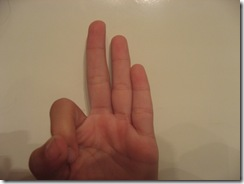# The Binary Number System – Part 2: Binary Weighting

In part 1, I illustrated how our decimal/Arabic number system is based on our ability to count up to nine with a single digit. After that, we add a digit (with a weighting of ten) and then continue to count the first digit from zero to nine again.

The best way to explain how computers count is to show an example. Let’s review again the way we count.

## Three versions of 3

When someone shows three fingers to us (with one finger and a thumb folded down), we interpret the number three:However, there are more ways of representing the same number:The reason is that we assign the same value to each finger. Any three fingers being up signifies the number three.

## All fingers are not created equal

The analogous counting system with computers is to assign different values to each finger. Look closely at the following picture (click on it if you have to). Youâ€™ll notice the numbers eight, four, two, and one on the fingers:

When we see a hand with fingers up, we add up the numbers on the finger. Some examples:Illustration A: The number fourteen (8+4+2)

## Putting it in numbers

So, here’s how computers represent each number above: They assign a 1 or a 0 to each finger position. If the finger is up, it’s assigned a 1. If the finger is down, it’s assigned a 0.

So example A (fourteen) would be represented as 1110. Example B above (five) would be represented as 0111. Example C would be represented as 1001.

Incidentally, the weighting of 8, 4, 2, 1 on each finger is called binary weighting: each finger has a value of twice the finger to the right of it. To complete the analogy, each finger represents one bit: one unit of binary information (a 1 or a 0). With four fingers/bits, we can represent any number between zero (all fingers down) to fifteen (Illustration A: all fingers up; 8+4+2+1).